# Python Program to Convert Hexadecimal to Octal

In this article, we've created some programs in Python, to convert hexadecimal number entered by user to its octal equivalent. Here are the list of programs:

• Hexadecimal to Octal using while Loop
• Using int() and oct() Methods
• Using user-defined Function
• Using Class and Object

Note - Before creating these programs, if you're not aware about steps used for the conversion, then refer to Hexadecimal to Octal Steps and Formula to get every required things.

## Hexadecimal to Octal using while Loop

To convert hexadecimal to octal number in Python, you have to ask from user to enter any number in hexadecimal number system, then convert that number into its octal equivalent as shown in the program given below:

```print("Enter the Hexadecimal Number: ")
hexdecnum = input()

chk = 0
decnum = 0
hexdecnumlen = len(hexdecnum)
hexdecnumlen = hexdecnumlen-1
i = 0
while hexdecnumlen>=0:
rem = hexdecnum[hexdecnumlen]
if rem>='0' and rem<='9':
rem = int(rem)
elif rem>='A' and rem<='F':
rem = ord(rem)
rem = rem-55
elif rem>='a' and rem<='f':
rem = ord(rem)
rem = rem-87
else:
chk = 1
break
decnum = decnum + (rem * (16 ** i))
hexdecnumlen = hexdecnumlen-1
i = i+1

if chk==0:
i = 0
octnum = []
while decnum!=0:
rem = decnum%8
octnum.insert(i, rem)
i = i+1
decnum = int(decnum/8)

print("\nEquivalent Octal Value is: ")
i = i-1
while i>=0:
print(octnum[i], end="")
i = i-1
print()
else:
print("\nInvalid Input!")```

Here is its sample run: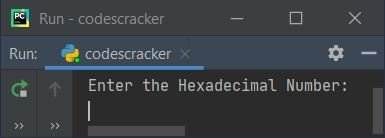Now supply the input say 23E5F as a hexadecimal number, then press `ENTER` key to convert and print its equivalent octal value as shown in the snapshot given below: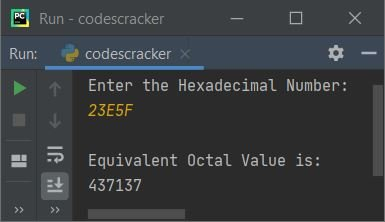In above program, the block of code inside while loop is used to convert given hexadecimal number to its equivalent decimal value. And the block of code inside if (if of if chk==0:) is used to convert decimal value to its equivalent octal value. That means, hexadecimal gets converted into its octal equivalent in indirect way.

Note - To get in-depth idea about the working of code used for hexadecimal to decimal conversion, refer to Python Hexadecimal to Decimal

Note - To get in-depth idea about the working of code used for decimal to octal conversion, refer to Decimal to Octal.

Note - You can also prefer, hexadecimal to binary, then binary to octal way to do the job. It is up to you.

#### Modified Version of Previous Program

This is the modified version of previous program, uses end= to skip printing of an automatic newline using print(). That is, using end, we've received the input in same line where the message Enter Hexadecimal Number: is printed:

```print("Enter Hexadecimal Number: ", end="")
hnum = input()

chk = dnum = i = 0
hlen = len(hnum) - 1
while hlen>=0:
if hnum[hlen]>='0' and hnum[hlen]<='9':
rem = int(hnum[hlen])
elif hnum[hlen]>='A' and hnum[hlen]<='F':
rem = ord(hnum[hlen]) - 55
elif hnum[hlen]>='a' and hnum[hlen]<='f':
rem = ord(hnum[hlen]) - 87
else:
chk = 1
break
dnum = dnum + (rem * (16 ** i))
hlen = hlen - 1
i = i+1

if chk==0:
i = 0
onum = []
while dnum != 0:
onum.insert(i, dnum % 8)
i = i + 1
dnum = int(dnum / 8)

print("\nEquivalent Octal Value = ", end="")
i = i - 1
while i >= 0:
print(onum[i], end="")
i = i - 1
print()
else:
print("\nInvalid Input!")```

Here is its sample run with hexadecimal number input 123F: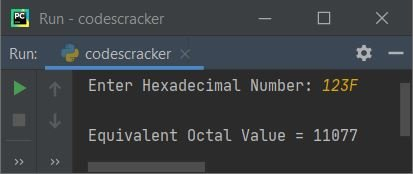## Hexadecimal to Octal using int() and oct()

This program uses int() and oct() methods to do the job. The int() method is used to return integer (decimal) equivalent of value passed as its argument. And the oct() method is used to return octal equivalent of value passed as its argument.

```print("Enter Hexadecimal Number: ", end="")
hnum = input()

onum = int(hnum, 16)
onum = oct(onum)
print("\nEquivalent Octal Value = ", onum)```

Here is its sample output after supplying 45EA as hexadecimal input: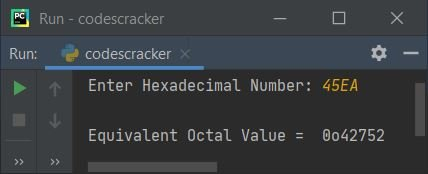Note - If you want to skip first two characters from octal number, just add [2:] after onum while printing. That is, replace the following statement:

`print("\nEquivalent Octal Value = ", onum)`

with the statement given below:

`print("\nEquivalent Octal Value = ", onum[2:])`

after replacing, now the output looks like after re-executing the program and supplying the same user input: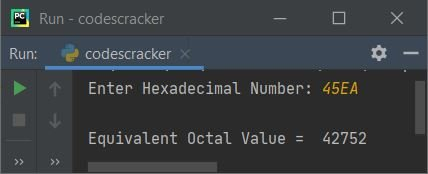## Hexadecimal to Octal using Function

Now this program is created using a user-defined function named HexToOct() and two predefined methods namely oct() and int() to do the same job as previous program has done.

```def HexToOct(h):
return oct(int(h, 16))

hnum = input()

onum = HexToOct(hnum)
print("\nEquivalent Octal Value = ", onum[2:])```

## Hexadecimal to Octal using Class

This is the last program of this article, created using a class named CodesCracker. That is, inside the class, we've defined a member function named HexToOct(). We have created an object named obj of this class to access its member function named HexToOct() using dot (.) operator.

```class CodesCracker:
def HexToOct(self, h):
return oct(int(h, 16))

hnum = input()

obj = CodesCracker()
onum = obj.HexToOct(hnum)
print("\nEquivalent Octal Value = ", onum[2:])```

#### Same Program in Other Languages

Python Online Test

« Previous Program Next Program »